Utilizamos tu perfil de LinkedIn y tus datos de actividad para personalizar los anuncios y mostrarte publicidad más relevante. Puedes cambiar tus preferencias de publicidad en cualquier momento.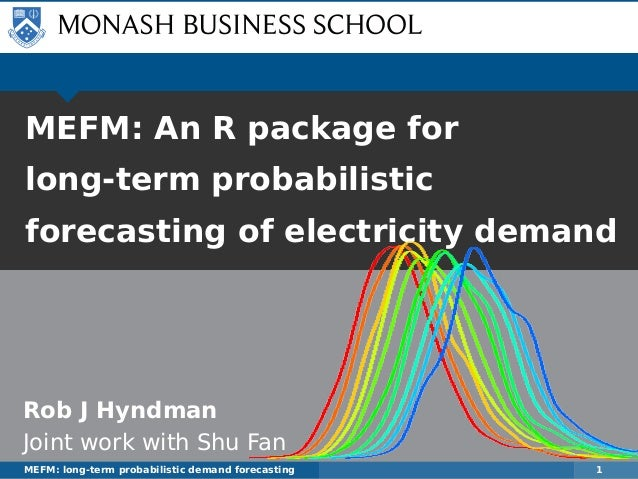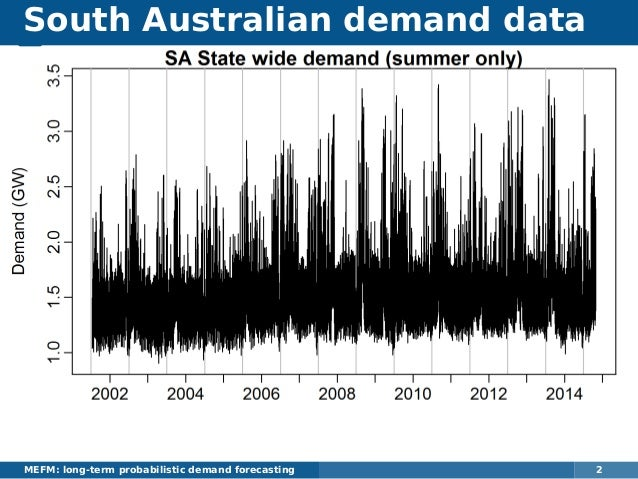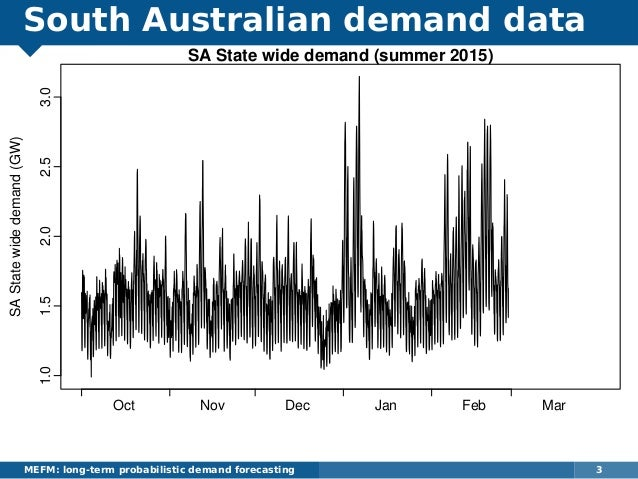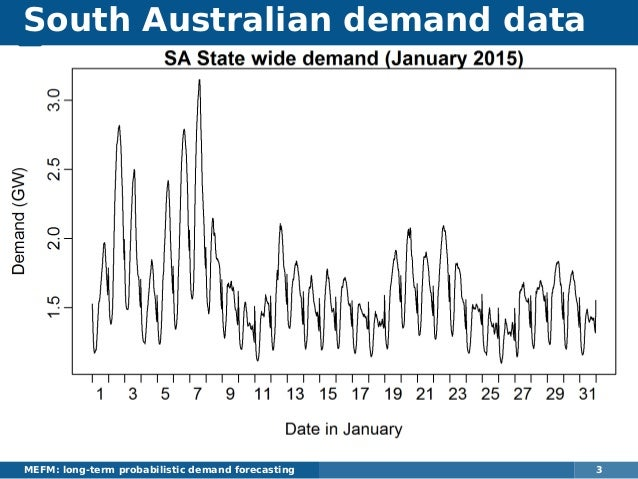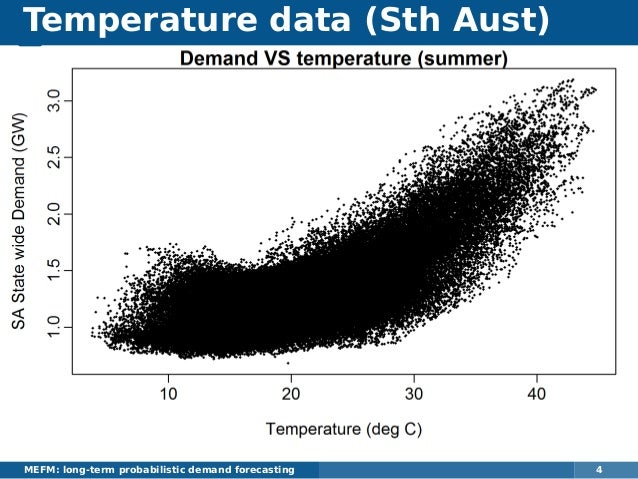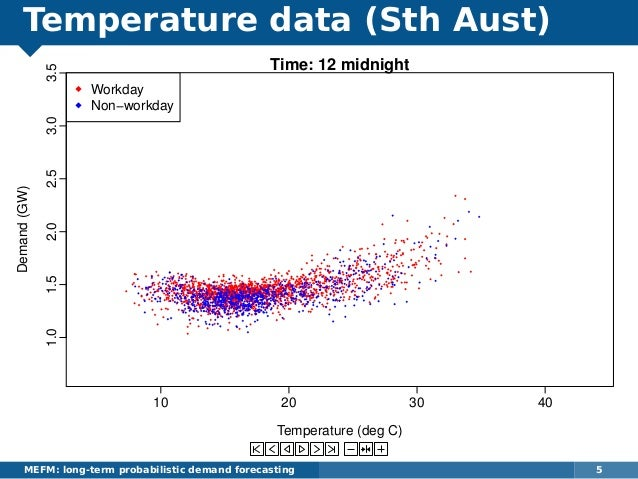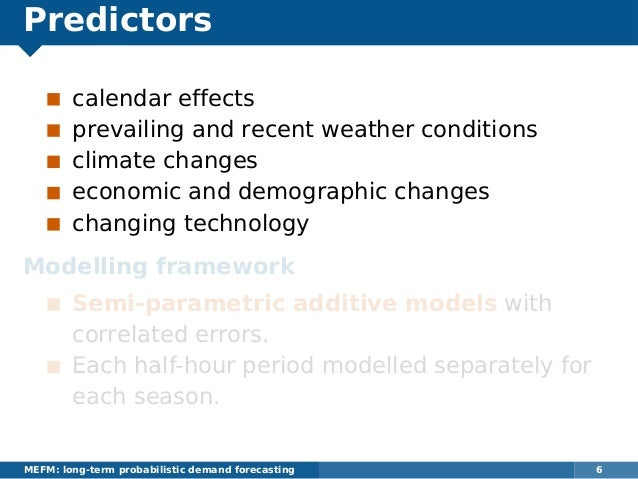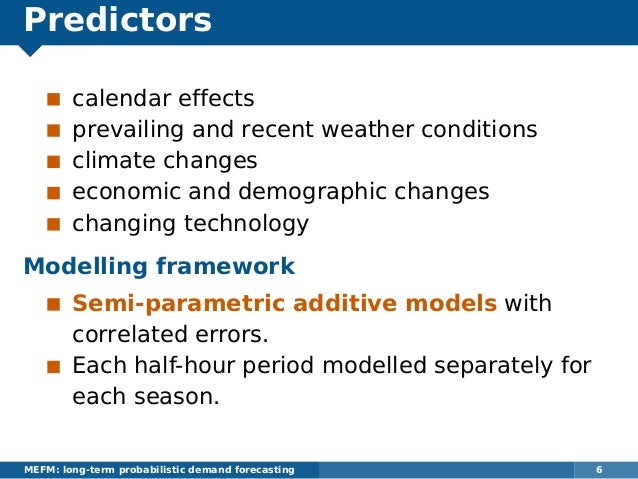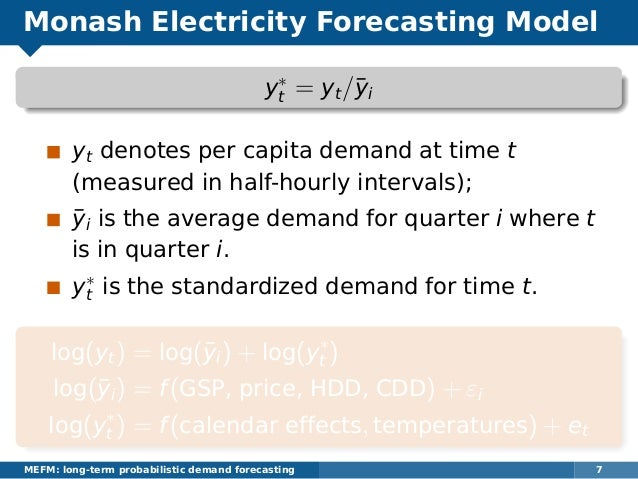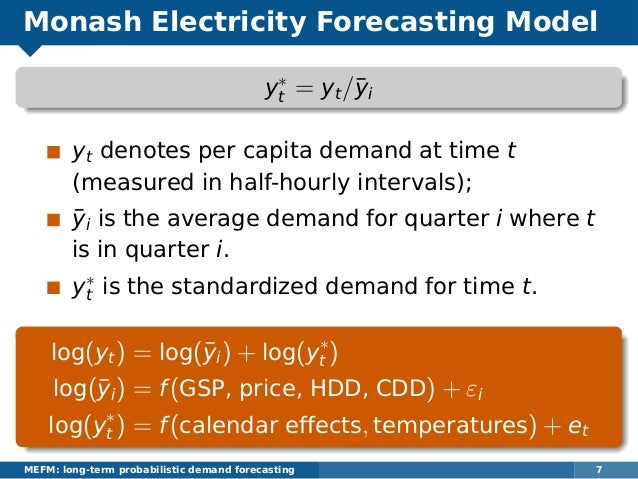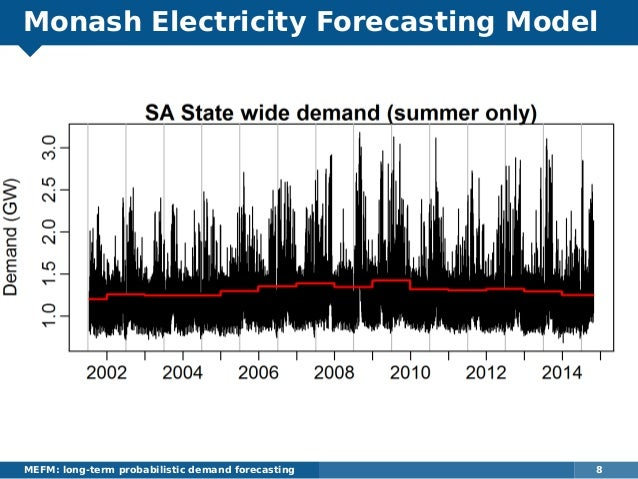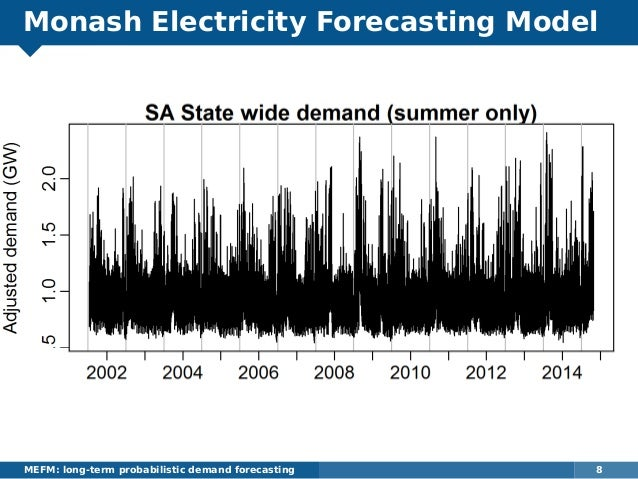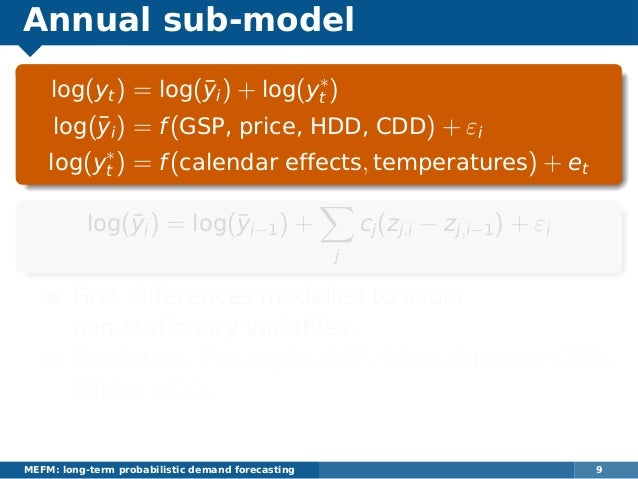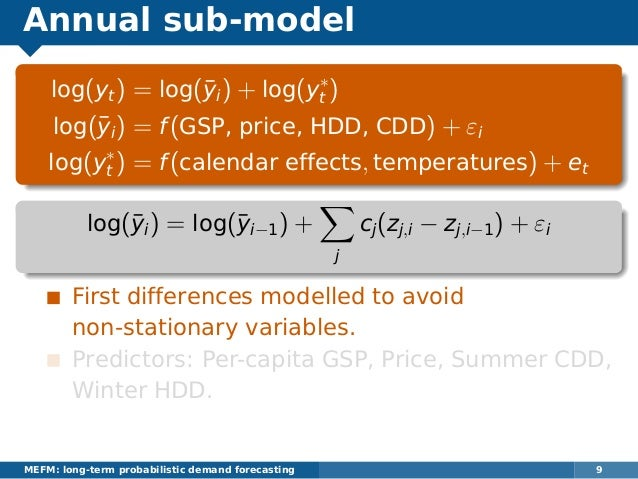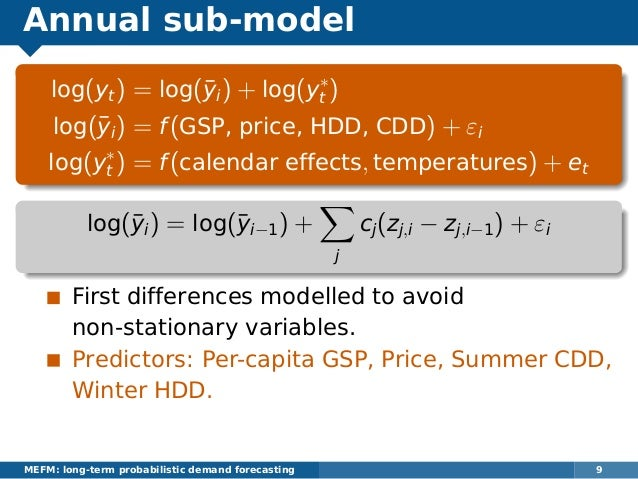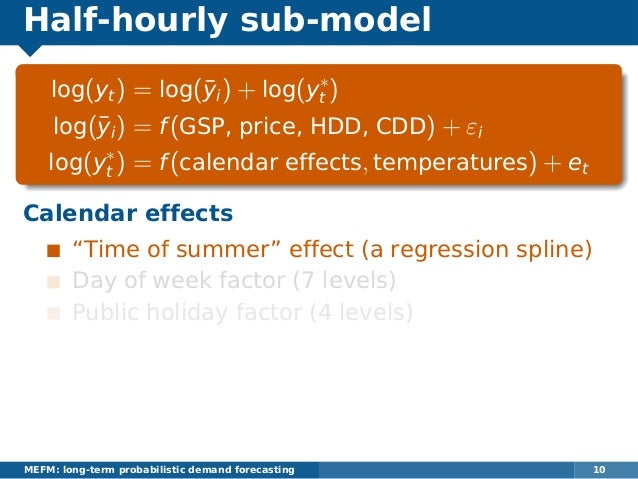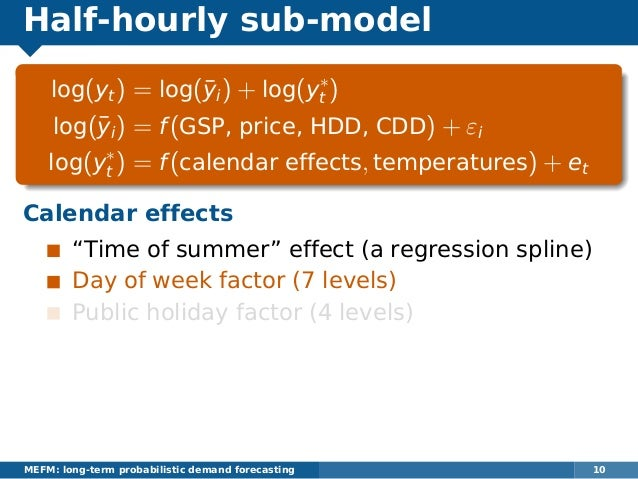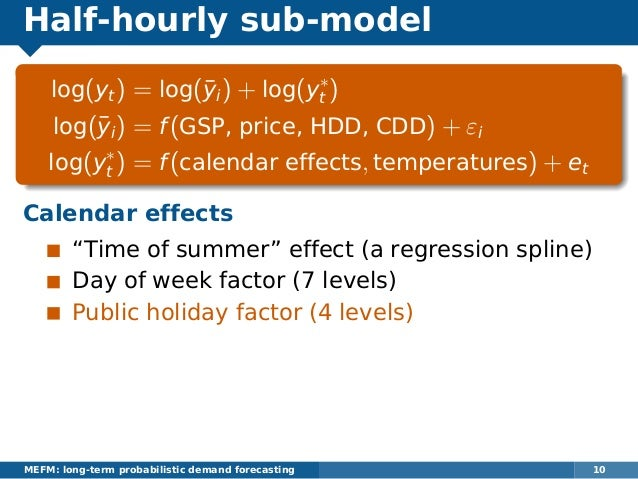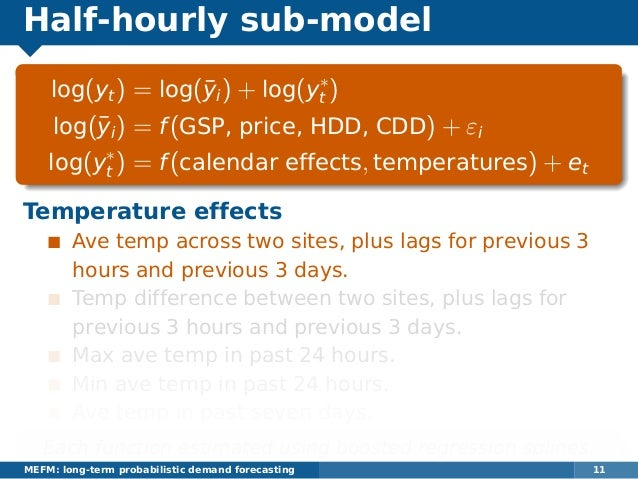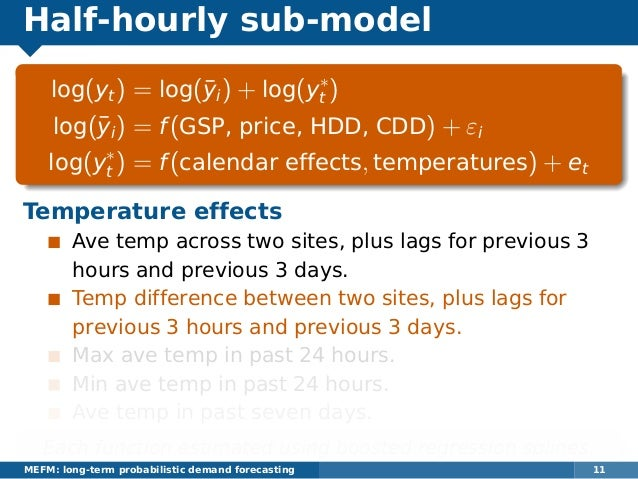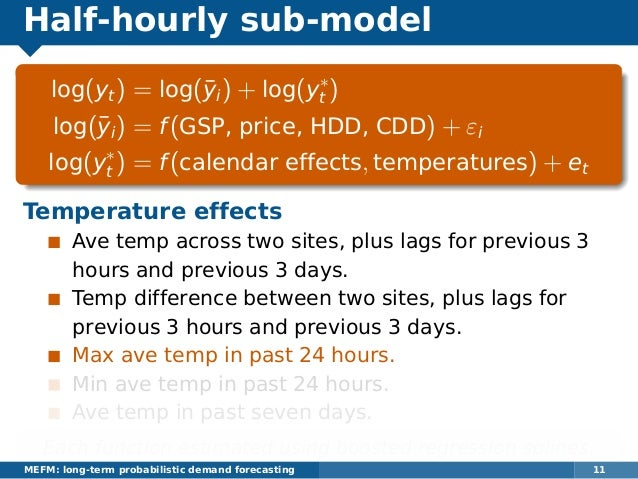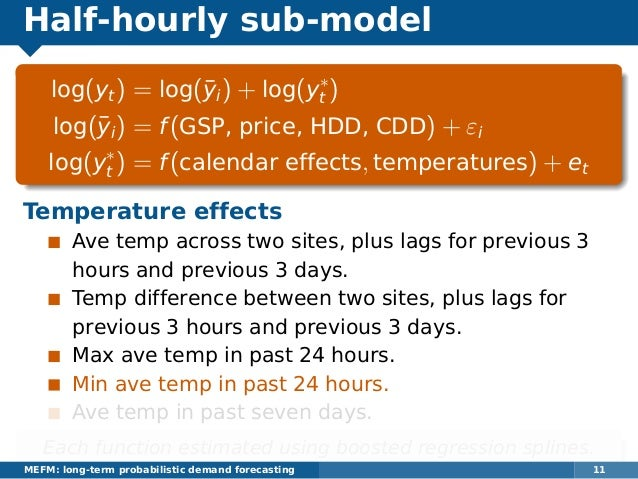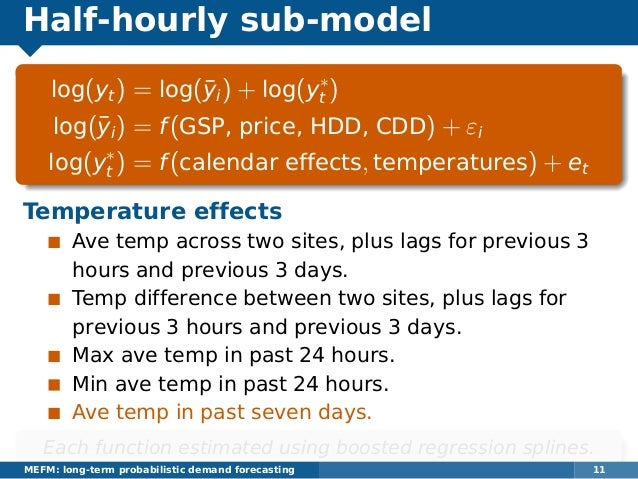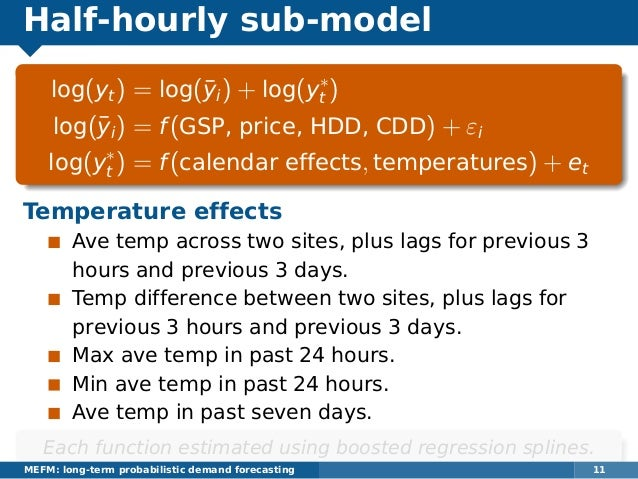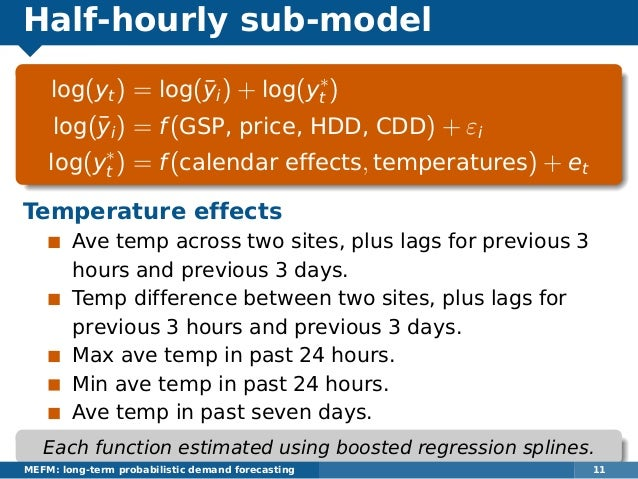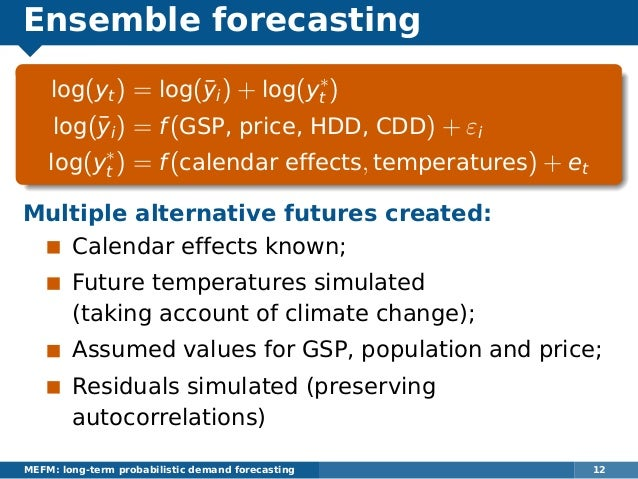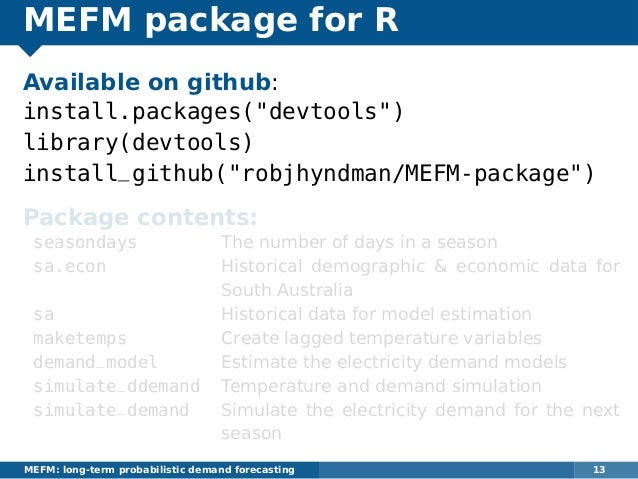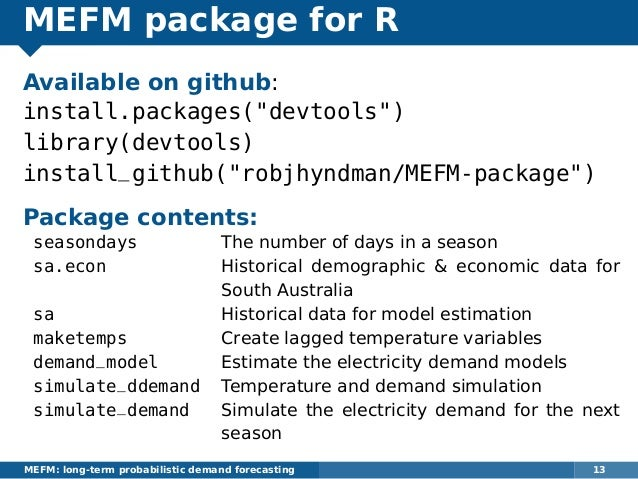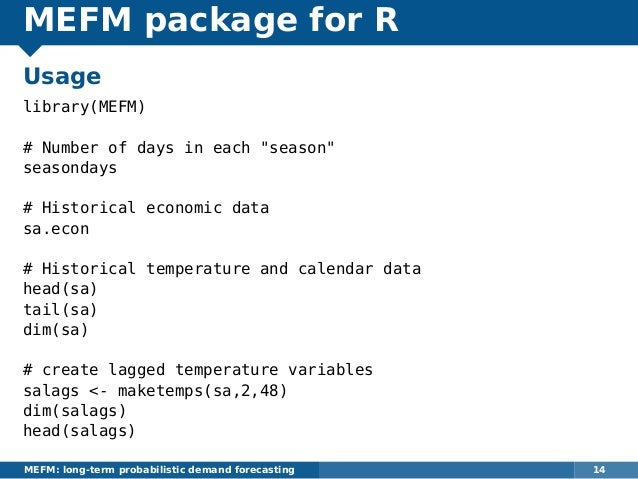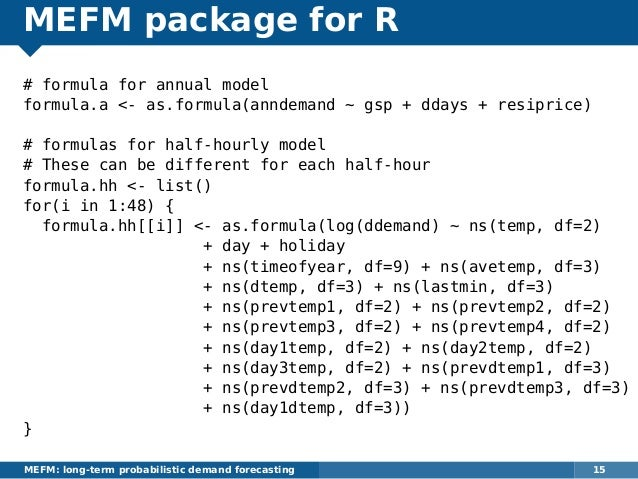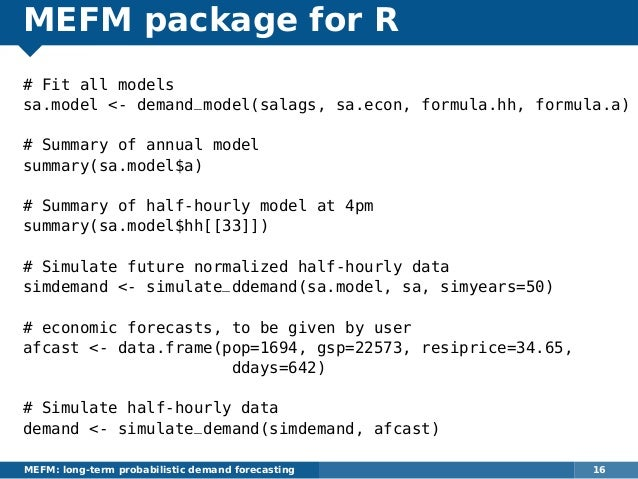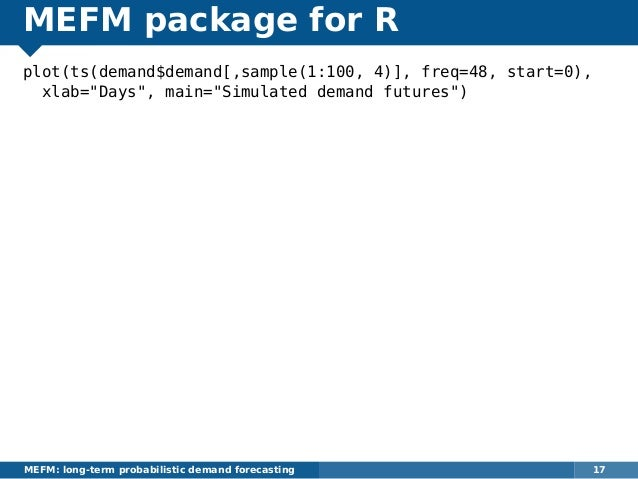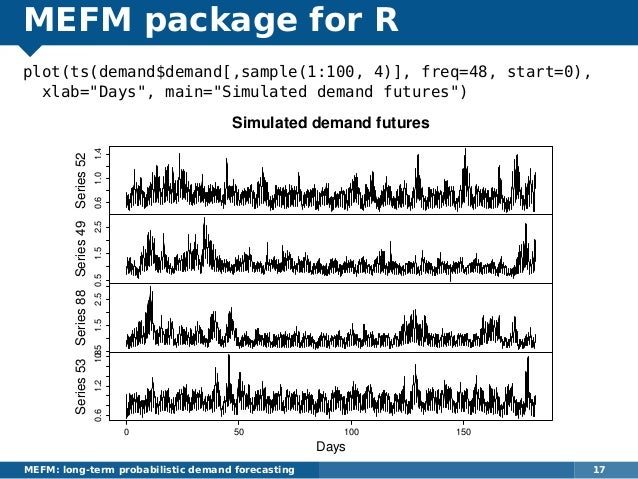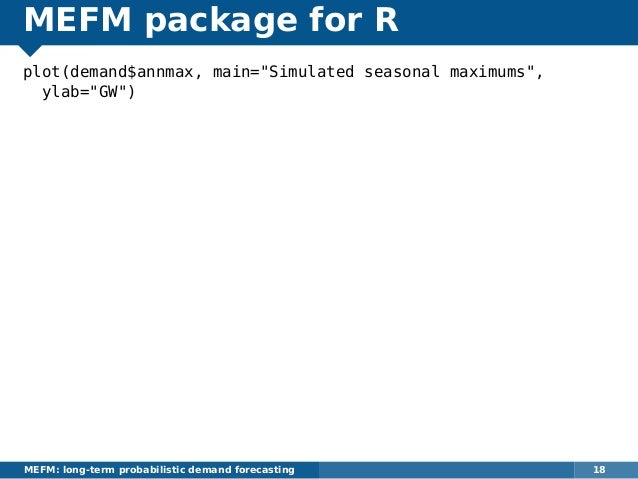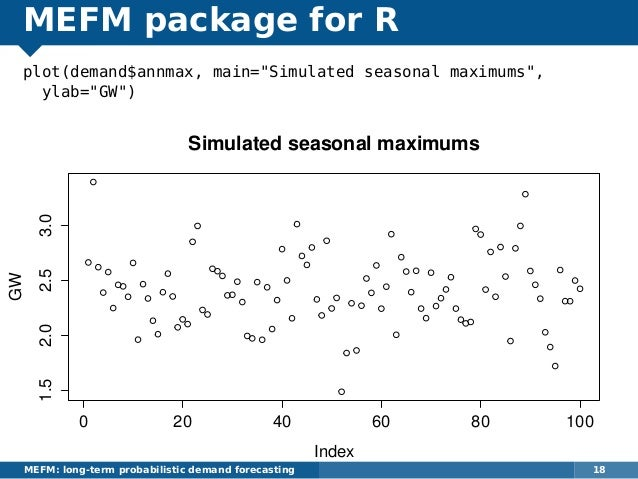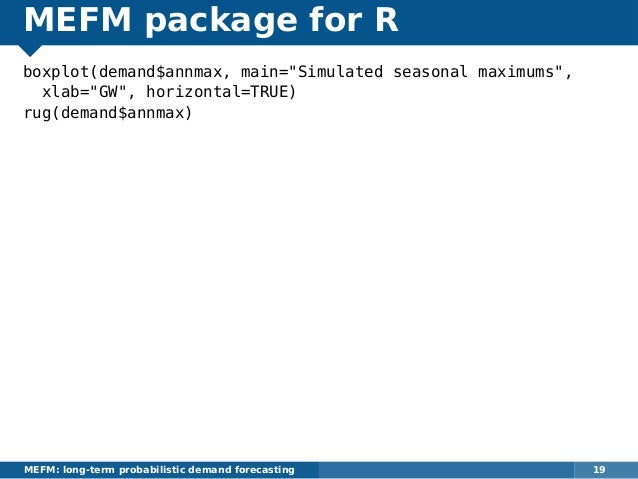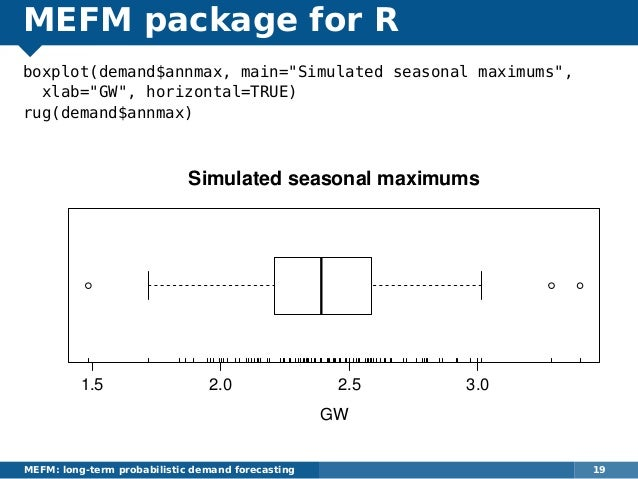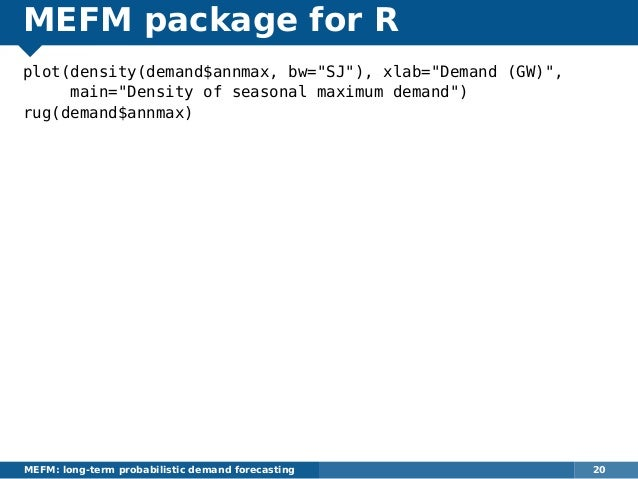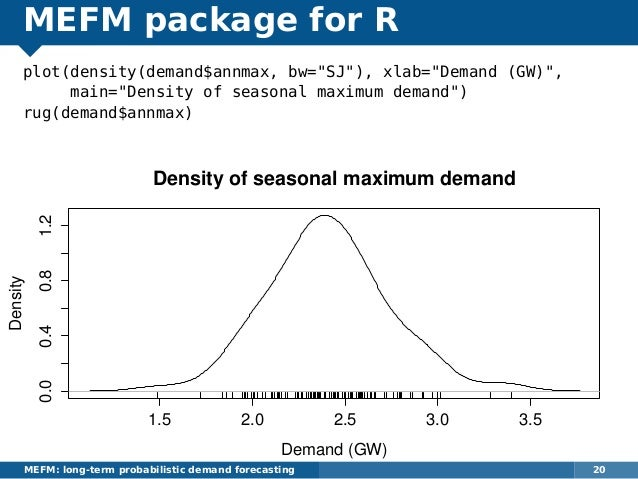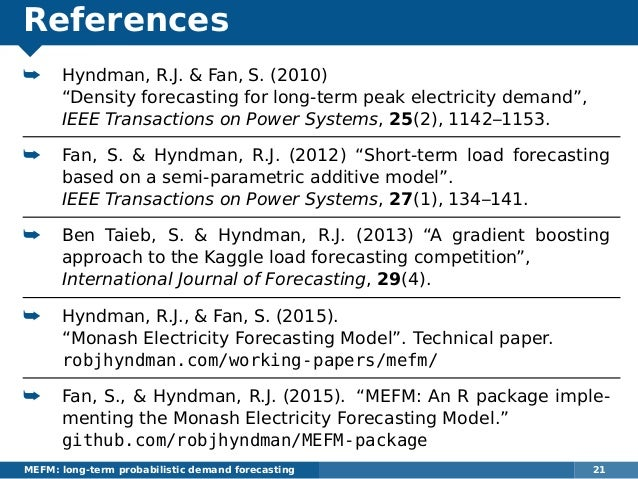Próxima SlideShare
Cargando en…5
×

# MEFM: An R package for long-term probabilistic forecasting of electricity demand

I will describe and demonstrate a new open-source R package that implements the Monash Electricity Forecasting Model, a semi-parametric probabilistic approach to forecasting long-term electricity demand. The underlying model proposed in Hyndman and Fan (2010) is now widely used in practice, particularly in Australia. The model has undergone many improvements and developments since it was first proposed, and these have been incorporated in this R implementation.

The package allows for ensemble forecasting of demand based on simulations of future sample paths of temperatures and other predictor variables. It requires the following data as inputs: half-hourly/hourly electricity demands; half-hourly/hourly temperatures at one or two locations; seasonal (e.g., quarterly) demographic and economic data; and public holiday data.

Peak electricity demand forecasting is important in medium and long-term planning of electricity supply. Extreme demand often leads to supply failure with consequential business and social disruption. Forecasting extreme demand events is therefore an important problem in energy management, and this package provides a useful tool for energy companies and regulators in future planning.

• Full Name
Comment goes here.

Are you sure you want to Yes No• Sé el primero en comentar

### MEFM: An R package for long-term probabilistic forecasting of electricity demand

1. 1. Rob J Hyndman Joint work with Shu Fan MEFM: long-term probabilistic demand forecasting 1 MEFM: An R package for long-term probabilistic forecasting of electricity demand
2. 2. South Australian demand data MEFM: long-term probabilistic demand forecasting 2
3. 3. South Australian demand data MEFM: long-term probabilistic demand forecasting 3 SA State wide demand (summer 2015) SAStatewidedemand(GW) 1.01.52.02.53.0 Oct Nov Dec Jan Feb Mar
4. 4. South Australian demand data MEFM: long-term probabilistic demand forecasting 3
5. 5. Temperature data (Sth Aust) MEFM: long-term probabilistic demand forecasting 4
6. 6. Temperature data (Sth Aust) MEFM: long-term probabilistic demand forecasting 5 10 20 30 40 1.01.52.02.53.03.5 Time: 12 midnight Temperature (deg C) Demand(GW) Workday Non−workday
7. 7. Predictors calendar effects prevailing and recent weather conditions climate changes economic and demographic changes changing technology Modelling framework Semi-parametric additive models with correlated errors. Each half-hour period modelled separately for each season. MEFM: long-term probabilistic demand forecasting 6
8. 8. Predictors calendar effects prevailing and recent weather conditions climate changes economic and demographic changes changing technology Modelling framework Semi-parametric additive models with correlated errors. Each half-hour period modelled separately for each season. MEFM: long-term probabilistic demand forecasting 6
9. 9. Monash Electricity Forecasting Model y∗ t = yt/¯yi yt denotes per capita demand at time t (measured in half-hourly intervals); ¯yi is the average demand for quarter i where t is in quarter i. y∗ t is the standardized demand for time t. log(yt) = log(¯yi) + log(y∗ t ) log(¯yi) = f(GSP, price, HDD, CDD) + εi log(y∗ t ) = f(calendar effects, temperatures) + et MEFM: long-term probabilistic demand forecasting 7
10. 10. Monash Electricity Forecasting Model y∗ t = yt/¯yi yt denotes per capita demand at time t (measured in half-hourly intervals); ¯yi is the average demand for quarter i where t is in quarter i. y∗ t is the standardized demand for time t. log(yt) = log(¯yi) + log(y∗ t ) log(¯yi) = f(GSP, price, HDD, CDD) + εi log(y∗ t ) = f(calendar effects, temperatures) + et MEFM: long-term probabilistic demand forecasting 7
11. 11. Monash Electricity Forecasting Model MEFM: long-term probabilistic demand forecasting 8
12. 12. Monash Electricity Forecasting Model MEFM: long-term probabilistic demand forecasting 8
13. 13. Annual sub-model log(yt) = log(¯yi) + log(y∗ t ) log(¯yi) = f(GSP, price, HDD, CDD) + εi log(y∗ t ) = f(calendar effects, temperatures) + et log(¯yi) = log(¯yi−1) + j cj(zj,i − zj,i−1) + εi First differences modelled to avoid non-stationary variables. Predictors: Per-capita GSP, Price, Summer CDD, Winter HDD. MEFM: long-term probabilistic demand forecasting 9
14. 14. Annual sub-model log(yt) = log(¯yi) + log(y∗ t ) log(¯yi) = f(GSP, price, HDD, CDD) + εi log(y∗ t ) = f(calendar effects, temperatures) + et log(¯yi) = log(¯yi−1) + j cj(zj,i − zj,i−1) + εi First differences modelled to avoid non-stationary variables. Predictors: Per-capita GSP, Price, Summer CDD, Winter HDD. MEFM: long-term probabilistic demand forecasting 9
15. 15. Annual sub-model log(yt) = log(¯yi) + log(y∗ t ) log(¯yi) = f(GSP, price, HDD, CDD) + εi log(y∗ t ) = f(calendar effects, temperatures) + et log(¯yi) = log(¯yi−1) + j cj(zj,i − zj,i−1) + εi First differences modelled to avoid non-stationary variables. Predictors: Per-capita GSP, Price, Summer CDD, Winter HDD. MEFM: long-term probabilistic demand forecasting 9
16. 16. Half-hourly sub-model log(yt) = log(¯yi) + log(y∗ t ) log(¯yi) = f(GSP, price, HDD, CDD) + εi log(y∗ t ) = f(calendar effects, temperatures) + et Calendar effects “Time of summer” effect (a regression spline) Day of week factor (7 levels) Public holiday factor (4 levels) MEFM: long-term probabilistic demand forecasting 10
17. 17. Half-hourly sub-model log(yt) = log(¯yi) + log(y∗ t ) log(¯yi) = f(GSP, price, HDD, CDD) + εi log(y∗ t ) = f(calendar effects, temperatures) + et Calendar effects “Time of summer” effect (a regression spline) Day of week factor (7 levels) Public holiday factor (4 levels) MEFM: long-term probabilistic demand forecasting 10
18. 18. Half-hourly sub-model log(yt) = log(¯yi) + log(y∗ t ) log(¯yi) = f(GSP, price, HDD, CDD) + εi log(y∗ t ) = f(calendar effects, temperatures) + et Calendar effects “Time of summer” effect (a regression spline) Day of week factor (7 levels) Public holiday factor (4 levels) MEFM: long-term probabilistic demand forecasting 10
19. 19. Half-hourly sub-model log(yt) = log(¯yi) + log(y∗ t ) log(¯yi) = f(GSP, price, HDD, CDD) + εi log(y∗ t ) = f(calendar effects, temperatures) + et Temperature effects Ave temp across two sites, plus lags for previous 3 hours and previous 3 days. Temp difference between two sites, plus lags for previous 3 hours and previous 3 days. Max ave temp in past 24 hours. Min ave temp in past 24 hours. Ave temp in past seven days. Each function estimated using boosted regression splines. MEFM: long-term probabilistic demand forecasting 11
20. 20. Half-hourly sub-model log(yt) = log(¯yi) + log(y∗ t ) log(¯yi) = f(GSP, price, HDD, CDD) + εi log(y∗ t ) = f(calendar effects, temperatures) + et Temperature effects Ave temp across two sites, plus lags for previous 3 hours and previous 3 days. Temp difference between two sites, plus lags for previous 3 hours and previous 3 days. Max ave temp in past 24 hours. Min ave temp in past 24 hours. Ave temp in past seven days. Each function estimated using boosted regression splines. MEFM: long-term probabilistic demand forecasting 11
21. 21. Half-hourly sub-model log(yt) = log(¯yi) + log(y∗ t ) log(¯yi) = f(GSP, price, HDD, CDD) + εi log(y∗ t ) = f(calendar effects, temperatures) + et Temperature effects Ave temp across two sites, plus lags for previous 3 hours and previous 3 days. Temp difference between two sites, plus lags for previous 3 hours and previous 3 days. Max ave temp in past 24 hours. Min ave temp in past 24 hours. Ave temp in past seven days. Each function estimated using boosted regression splines. MEFM: long-term probabilistic demand forecasting 11
22. 22. Half-hourly sub-model log(yt) = log(¯yi) + log(y∗ t ) log(¯yi) = f(GSP, price, HDD, CDD) + εi log(y∗ t ) = f(calendar effects, temperatures) + et Temperature effects Ave temp across two sites, plus lags for previous 3 hours and previous 3 days. Temp difference between two sites, plus lags for previous 3 hours and previous 3 days. Max ave temp in past 24 hours. Min ave temp in past 24 hours. Ave temp in past seven days. Each function estimated using boosted regression splines. MEFM: long-term probabilistic demand forecasting 11
23. 23. Half-hourly sub-model log(yt) = log(¯yi) + log(y∗ t ) log(¯yi) = f(GSP, price, HDD, CDD) + εi log(y∗ t ) = f(calendar effects, temperatures) + et Temperature effects Ave temp across two sites, plus lags for previous 3 hours and previous 3 days. Temp difference between two sites, plus lags for previous 3 hours and previous 3 days. Max ave temp in past 24 hours. Min ave temp in past 24 hours. Ave temp in past seven days. Each function estimated using boosted regression splines. MEFM: long-term probabilistic demand forecasting 11
24. 24. Half-hourly sub-model log(yt) = log(¯yi) + log(y∗ t ) log(¯yi) = f(GSP, price, HDD, CDD) + εi log(y∗ t ) = f(calendar effects, temperatures) + et Temperature effects Ave temp across two sites, plus lags for previous 3 hours and previous 3 days. Temp difference between two sites, plus lags for previous 3 hours and previous 3 days. Max ave temp in past 24 hours. Min ave temp in past 24 hours. Ave temp in past seven days. Each function estimated using boosted regression splines. MEFM: long-term probabilistic demand forecasting 11
25. 25. Half-hourly sub-model log(yt) = log(¯yi) + log(y∗ t ) log(¯yi) = f(GSP, price, HDD, CDD) + εi log(y∗ t ) = f(calendar effects, temperatures) + et Temperature effects Ave temp across two sites, plus lags for previous 3 hours and previous 3 days. Temp difference between two sites, plus lags for previous 3 hours and previous 3 days. Max ave temp in past 24 hours. Min ave temp in past 24 hours. Ave temp in past seven days. Each function estimated using boosted regression splines. MEFM: long-term probabilistic demand forecasting 11
26. 26. Ensemble forecasting log(yt) = log(¯yi) + log(y∗ t ) log(¯yi) = f(GSP, price, HDD, CDD) + εi log(y∗ t ) = f(calendar effects, temperatures) + et Multiple alternative futures created: Calendar effects known; Future temperatures simulated (taking account of climate change); Assumed values for GSP, population and price; Residuals simulated (preserving autocorrelations) MEFM: long-term probabilistic demand forecasting 12
27. 27. MEFM package for R Available on github: install.packages("devtools") library(devtools) install_github("robjhyndman/MEFM-package") Package contents: seasondays The number of days in a season sa.econ Historical demographic & economic data for South Australia sa Historical data for model estimation maketemps Create lagged temperature variables demand_model Estimate the electricity demand models simulate_ddemand Temperature and demand simulation simulate_demand Simulate the electricity demand for the next season MEFM: long-term probabilistic demand forecasting 13
28. 28. MEFM package for R Available on github: install.packages("devtools") library(devtools) install_github("robjhyndman/MEFM-package") Package contents: seasondays The number of days in a season sa.econ Historical demographic & economic data for South Australia sa Historical data for model estimation maketemps Create lagged temperature variables demand_model Estimate the electricity demand models simulate_ddemand Temperature and demand simulation simulate_demand Simulate the electricity demand for the next season MEFM: long-term probabilistic demand forecasting 13
29. 29. MEFM package for R Usage library(MEFM) # Number of days in each "season" seasondays # Historical economic data sa.econ # Historical temperature and calendar data head(sa) tail(sa) dim(sa) # create lagged temperature variables salags <- maketemps(sa,2,48) dim(salags) head(salags) MEFM: long-term probabilistic demand forecasting 14
30. 30. MEFM package for R # formula for annual model formula.a <- as.formula(anndemand ~ gsp + ddays + resiprice) # formulas for half-hourly model # These can be different for each half-hour formula.hh <- list() for(i in 1:48) { formula.hh[[i]] <- as.formula(log(ddemand) ~ ns(temp, df=2) + day + holiday + ns(timeofyear, df=9) + ns(avetemp, df=3) + ns(dtemp, df=3) + ns(lastmin, df=3) + ns(prevtemp1, df=2) + ns(prevtemp2, df=2) + ns(prevtemp3, df=2) + ns(prevtemp4, df=2) + ns(day1temp, df=2) + ns(day2temp, df=2) + ns(day3temp, df=2) + ns(prevdtemp1, df=3) + ns(prevdtemp2, df=3) + ns(prevdtemp3, df=3) + ns(day1dtemp, df=3)) } MEFM: long-term probabilistic demand forecasting 15
31. 31. MEFM package for R # Fit all models sa.model <- demand_model(salags, sa.econ, formula.hh, formula.a) # Summary of annual model summary(sa.model\$a) # Summary of half-hourly model at 4pm summary(sa.model\$hh[]) # Simulate future normalized half-hourly data simdemand <- simulate_ddemand(sa.model, sa, simyears=50) # economic forecasts, to be given by user afcast <- data.frame(pop=1694, gsp=22573, resiprice=34.65, ddays=642) # Simulate half-hourly data demand <- simulate_demand(simdemand, afcast) MEFM: long-term probabilistic demand forecasting 16
32. 32. MEFM package for R plot(ts(demand\$demand[,sample(1:100, 4)], freq=48, start=0), xlab="Days", main="Simulated demand futures") MEFM: long-term probabilistic demand forecasting 17
33. 33. MEFM package for R plot(ts(demand\$demand[,sample(1:100, 4)], freq=48, start=0), xlab="Days", main="Simulated demand futures")0.61.01.4 Series52 0.51.52.5 Series49 0.51.52.5 Series88 0.61.21.8 0 50 100 150 Series53 Days Simulated demand futures MEFM: long-term probabilistic demand forecasting 17
34. 34. MEFM package for R plot(demand\$annmax, main="Simulated seasonal maximums", ylab="GW") MEFM: long-term probabilistic demand forecasting 18
35. 35. MEFM package for R plot(demand\$annmax, main="Simulated seasonal maximums", ylab="GW") 0 20 40 60 80 100 1.52.02.53.0 Simulated seasonal maximums Index GW MEFM: long-term probabilistic demand forecasting 18
36. 36. MEFM package for R boxplot(demand\$annmax, main="Simulated seasonal maximums", xlab="GW", horizontal=TRUE) rug(demand\$annmax) MEFM: long-term probabilistic demand forecasting 19
37. 37. MEFM package for R boxplot(demand\$annmax, main="Simulated seasonal maximums", xlab="GW", horizontal=TRUE) rug(demand\$annmax) 1.5 2.0 2.5 3.0 Simulated seasonal maximums GW MEFM: long-term probabilistic demand forecasting 19
38. 38. MEFM package for R plot(density(demand\$annmax, bw="SJ"), xlab="Demand (GW)", main="Density of seasonal maximum demand") rug(demand\$annmax) MEFM: long-term probabilistic demand forecasting 20
39. 39. MEFM package for R plot(density(demand\$annmax, bw="SJ"), xlab="Demand (GW)", main="Density of seasonal maximum demand") rug(demand\$annmax) 1.5 2.0 2.5 3.0 3.5 0.00.40.81.2 Density of seasonal maximum demand Demand (GW) Density MEFM: long-term probabilistic demand forecasting 20
40. 40. References ¯ Hyndman, R.J. & Fan, S. (2010) “Density forecasting for long-term peak electricity demand”, IEEE Transactions on Power Systems, 25(2), 1142–1153. ¯ Fan, S. & Hyndman, R.J. (2012) “Short-term load forecasting based on a semi-parametric additive model”. IEEE Transactions on Power Systems, 27(1), 134–141. ¯ Ben Taieb, S. & Hyndman, R.J. (2013) “A gradient boosting approach to the Kaggle load forecasting competition”, International Journal of Forecasting, 29(4). ¯ Hyndman, R.J., & Fan, S. (2015). “Monash Electricity Forecasting Model”. Technical paper. robjhyndman.com/working-papers/mefm/ ¯ Fan, S., & Hyndman, R.J. (2015). “MEFM: An R package imple- menting the Monash Electricity Forecasting Model.” github.com/robjhyndman/MEFM-package MEFM: long-term probabilistic demand forecasting 21

### Inicia sesión para ver los comentarios

•#### Puindi

Sep. 20, 2015
•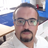#### mathematixy

Dec. 12, 2017
•#### LaikangbamAneka

Aug. 20, 2020

#### Vistas

Total de vistas

3.774

En Slideshare

0

De embebidos

0

Número de embebidos

1.857

Descargas

84

Compartidos

0

Comentarios

0

Me gusta

3